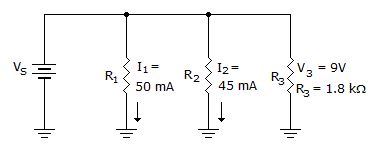# Electronics - Parallel Circuits - Discussion

Discussion Forum : Parallel Circuits - General Questions (Q.No. 29)
29.What does RT equal in the given circuit?

901802001.8 kExplanation:
No answer description is available. Let's discuss.
Discussion:
8 comments Page 1 of 1.

Naveen kumar said:   5 years ago
Thank you @Prashant.

Narasayya said:   5 years ago
R1=9/50,R2=9/45,R3=18/10,
1/RT=5/9(10+9+1),
1/RT=5/9X20=100/9,
RT=9/100X1000=90ohms.

Prashant said:   6 years ago
R1 = 180 ohm and R2= 200 ohm.
In a parallel circuit, the total res is less than lowest value res.

So answer is 90 because lowest res is r1 180 ohm and in options, less than 180 is only 90 ohms.

Nisha said:   7 years ago
r = v/I.
r1 = 9/50m = 180ohm.
r2 = 9/45m = 200ohm.
r3 = 1.8kohm.

1/Rt = 1/r1 + 1/r2 + 1/r3 = 90ohm.

V=9v.

I3=9/1.8k=5mA.

Itotal=50+5+45=100mA.

Req=V/I=9/(100 x 10-3).

=90ohm.

I3= V3/R3= 9/1.8= 5mA
Total Current= I1+I2+I3= 100mA
Rt= V/I= 9/100= 0.09kohm= 90 ohm ;)

Naseem Ul Aziz said:   1 decade ago
r1=9/50ma=180 ohm
r2=9/45ma=200 ohm
r3=1800 ohm
1/rt=(1/180)+(1/200)+(1/1800)=0.00555+0.005+0.00055=0.0111=90 ohm